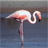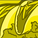# QlikView App Dev

Discussion Board for collaboration related to QlikView App Development.

Announcements
cancel
Showing results for
Did you mean:Partner

## How to ignore selection when using Rank and Aggr?

Hi,

I create a chart that rank the Top Operator of every Region and I only want to show the Top Operator ID.  It's done using Rank and Aggr

aggr(if( aggr(rank(sum({\$<CLIENT_REGION_NAME= >}AMOUNT)),CLIENT_REGION_NAME,[OPERATOR ID]) = 1,
avg({\$<CLIENT_REGION_NAME= >}[OPERATOR ID])),CLIENT_REGION_NAME,[OPERATOR ID])

My Question is, if I would like to ignore the selection of OPERATOR ID, meaning when someone select an Operator, the chart should not change.  How should I do it?  I tried to put OPERATOR= in the set analysis but it shows only the region which the OPERATOR selected.  I do not what anything change in the chart but couldn't find a solution.

Please find example in the attachment file

1 Solution

Accepted SolutionsMVP

May be this

Only({<OPERATOR, [OPERATOR ID]>}Aggr(If(Rank(Sum({\$<CLIENT_REGION_NAME, OPERATOR, [OPERATOR ID]>}AMOUNT)) = 1,

Avg({\$<CLIENT_REGION_NAME, OPERATOR, [OPERATOR ID]>}[OPERATOR ID])), CLIENT_REGION_NAME, [OPERATOR ID]))

5 RepliesMVP

May be this

Only({<OPERATOR, [OPERATOR ID]>}Aggr(If(Rank(Sum({\$<CLIENT_REGION_NAME, OPERATOR, [OPERATOR ID]>}AMOUNT)) = 1,

Avg({\$<CLIENT_REGION_NAME, OPERATOR, [OPERATOR ID]>}[OPERATOR ID])), CLIENT_REGION_NAME, [OPERATOR ID]))Partner
Author

Thank you very much! It works!!Creator

Hi Sunny

I try to create a calculated dimension and face the same problem as Chia. For some reason, I'm not able to adapt your solution properly to my situation, so I hope you will help me out.

I want to calculate the last 10 days available in my data for an order. So the formula I use for getting the Dates is as below:

Only({<DATE_NO, REP_DATE, ID_DATE={"<\$(=Date(Floor(Now(),1),'YYYY-MM-DD'))"}>}

Aggr({<DATE_NO, REP_DATE, ID_DATE={"<\$(=Date(Floor(Now(),1),'YYYY-MM-DD'))"}>} NoDistinct

If((Rank(Only({<DATE_NO, REP_DATE, ID_DATE={"<\$(=Date(Floor(Now(),1),'YYYY-MM-DD'))"}>}ID_DATE))<=10),

Dual(ID_DATE,Rank(ID_DATE)),

Null()

),

(ID_DATE,(NUMERIC,DECENDING))

)

)

However, if I select a date, the Dimension is reduced to the selected date and does not show the last 10 days anymore.

Do you have any suggestions, what's wrong?

Thank you very much for your help and best regards,

DirkMVP

May be try this

Only({<DATE_NO, REP_DATE, ID_DATE={"<\$(=Date(Floor(Now(),1),'YYYY-MM-DD'))"}>}

Aggr({<DATE_NO, REP_DATE, ID_DATE={"<\$(=Date(Floor(Now(),1),'YYYY-MM-DD'))"}>} NoDistinct

If((Rank(Only({<DATE_NO, REP_DATE, ID_DATE={"<\$(=Date(Floor(Now(),1),'YYYY-MM-DD'))"}>}ID_DATE))<=10),

Dual(Only({<DATE_NO, REP_DATE, ID_DATE={"<\$(=Date(Floor(Now(),1),'YYYY-MM-DD'))"}>} ID_DATE), Rank(Only({<DATE_NO, REP_DATE, ID_DATE={"<\$(=Date(Floor(Now(),1),'YYYY-MM-DD'))"}>} ID_DATE))),

Null()

),

(ID_DATE,(NUMERIC,DECENDING))

)

)Creator

Hi Sunny,

thank's a lot. Together with a small modification in my measure, this did the trick.